## Titration

### Learning Objectives

• Define equivalence point.
• Describe how to perform a titration experiment.
• Perform calculations to determine concentration of unknown acid or base.
• Describe titration curves of acid-base neutralization reactions.

## Titration Experiment

### Didn’t that used to be French fries?

A lot of research is going on these days involving the development of biodiesel fuels. Often this material can be made from used vegetable oils. The vegetable oil is treated with lye to create the biofuel. In the oils is a variable amount of acid that needs to be determined so the workers will know how much lye to add to make the final fuel. Before the lye is added, the native vegetable oil is titrated to find out how much free acid is present. Then the amount of lye added can be adjusted to take into account the amount needed to neutralize these free acids.In the neutralization of hydrochloric acid by sodium hydroxide, the mole ratio of acid to base is 1:1.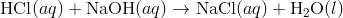One mole of HCl would be fully neutralized by one mole of NaOH. If instead the hydrochloric acid was reacted with barium hydroxide, the mole ratio would be 2:1.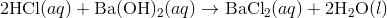Now two moles of HCl would be required to neutralize one mole of Ba(OH) 2 . The mole ratio insures that the number of moles of H + ions supplied by the acid is equal to the number of OH ions supplied by the base. This must be the case for neutralization to occur. The equivalence point is the point in a neutralization reaction where the number of moles of hydrogen ions is equal to the number of moles of hydroxide ions.

In the laboratory, it is useful to have an experiment where the unknown concentration of an acid or a base can be determined. This can be accomplished by performing a controlled neutralization reaction. A titration is an experiment where a volume of a solution of known concentration is added to a volume of another solution in order to determine its concentration. Many titrations are acid-base neutralization reactions, though other types of titrations can also be performed.

In order to perform an acid-base titration, the chemist must have a way to visually detect that the neutralization reaction has occurred. An indicator is a substance that has a distinctly different color when in an acidic or basic solution. A commonly used indicator for strong acid-strong base titrations is phenolphthalein. Solutions in which a few drops of phenolphthalein have been added turn from colorless to brilliant pink as the solution turns from acidic to basic. The steps in a titration reaction are outlined below.

1. A measured volume of an acid of unknown concentration is added to an Erlenmeyer flask.
2. Several drops of an indicator are added to the acid and mixed by swirling the flask.
3. A buret is filled with the base solution of known molarity.
4. The stopcock of the buret is opened and base is slowly added to the acid while the flask is constantly swirled to insure mixing. The stopcock is closed at the exact point at which the indicator just changes color.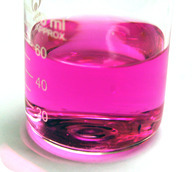Figure 1. Phenolphthalein in basic solution.

The standard solution is the solution in a titration whose concentration is known. In the titration described above the base solution is the standard solution. It is very important in a titration to add the solution from the buret slowly so that the point at which the indicator changes color can be found accurately.

The end point of a titration is the point at which the indicator changes color. When phenolphthalein is the indicator, the end point will be signified by a faint pink color.

## Titration CalculationsThe manufacture of soap requires a number of chemistry techniques. One necessary piece of information is the saponification number. This is the amount of base needed to hydrolyze a certain amount of fat to produce the free fatty acids that are an essential part of the final product.

The fat is heated with a known amount of base (usually NaOH or KOH). After hydrolysis is complete, the left-over base is titrated to determine how much was needed to hydrolyze the fat sample.

### Titration Calculations

At the equivalence point in a neutralization, the moles of acid are equal to the moles of base.

moles acid = moles base

Recall that the molarity (M) of a solution is defined as the moles of the solute divided by the liters of solution (L). So the moles of solute are therefore equal to the molarity of a solution multiplied by the volume in liters.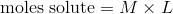We can then set the moles of acid equal to the moles of base.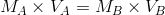is the molarity of the acid, while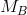is the molarity of the base.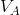and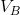are the volumes of the acid and base, respectively.

Suppose that a titration is performed and 20.70 mL of 0.500 M NaOH is required to reach the end point when titrated against 15.00 mL of HCl of unknown concentration. The above equation can be used to solve for the molarity of the acid.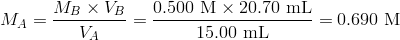The higher molarity of the acid compared to the base in this case means that a smaller volume of the acid is required to reach the equivalence point.

The above equation works only for neutralizations in which there is a 1:1 ratio between the acid and the base. The sample problem below demonstrates the technique to solve a titration problem for a titration of sulfuric acid with sodium hydroxide.

#### Sample Problem: Titration

In a titration of sulfuric acid against sodium hydroxide, 32.20 mL of 0.250 M NaOH is required to neutralize 26.60 mL of H2SO4. Calculate the molarity of the sulfuric acid.

Step 1: List the known values and plan the problem.

Known

• molarity NaOH = 0.250 M
• volume NaOH = 32.20 mL
• volume H 2 SO 4 = 26.60 mL

Unkonwn

• molarity H 2 SO 4 = ?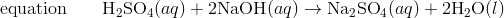First determine the moles of NaOH in the reaction. From the mole ratio, calculate the moles of H 2 SO 4 that reacted. Finally, divide the moles H 2 SO 4 by its volume to get the molarity.

Step 2: Solve.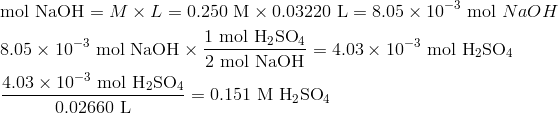The volume of H2SO4 required is smaller than the volume of NaOH because of the two hydrogen ions contributed by each molecule.

## Titration Curves

### Where did graphs come from?

The  x–y plot that we know of as a graph was the brainchild of the French mathematician-philosopher Rene Descartes (1596–1650). His studies in mathematics led him to develop what was known as “Cartesian geometry,” including the idea of our current graphs. The coordinates are often referred to as Cartesian coordinates.### Titration Curves

As base is added to acid at the beginning of a titration, the pH rises very slowly. Nearer to the equivalence point, the pH begins to rapidly increase. If the titration is a strong acid with a strong base, the pH at the equivalence point is equal to 7. A bit past the equivalence point, the rate of change of the pH again slows down. A titration curve is a graphical representation of the pH of a solution during a titration. The Figure below shows two different examples of a strong acid-strong base titration curve. On the left is a titration in which the base is added to the acid and so the pH progresses from low to high. On the right is a titration in which the acid is added to the base. In this case, the pH starts out high and decreases during the titration. In both cases, the equivalence point is reached when the moles of acid and base are equal and the pH is 7. This also corresponds to the color change of the indicator.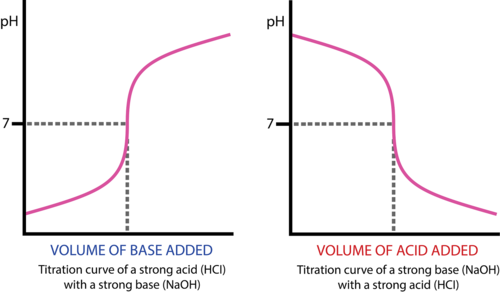Figure 2. A titration curve shows the pH changes that occur during the titration of an acid with a base. On the left, base is being added to acid. On the right, acid is being added to base. In both cases, the equivalence point is at pH 7.

Titration curves can also be generated in the case of a weak acid-strong base titration or a strong base-weak acid titration. The general shape of the titration curve is the same, but the pH at the equivalence point is different. In a weak acid-strong base titration, the pH is greater than 7 at the equivalence point. In a strong acid-weak base titration, the pH is less than 7 at the equivalence point.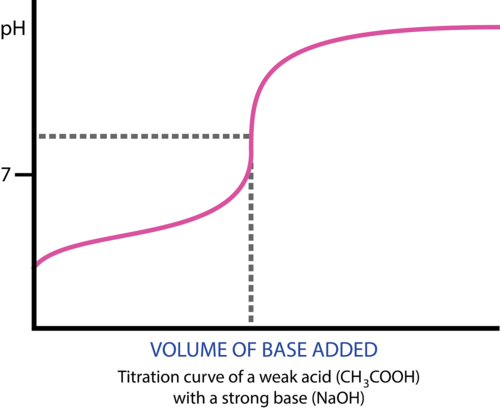Figure 3. Titration curve of weak acid and strong base.

### Summary

• Definitions are given for equivalence point, titration and indicator.
• The process for carrying out a titration is described.
• The process of calculating concentration from titration data is described and illustrated.
• Acid-base titration curves are described.

### Practice

#### Titration Experiment

Watch the video at the link below and answer the following questions:

1. What is the indicator used?
2. What color is it in acid solution?
3. What is the glass tube called that contains the known concentration of sodium hydroxide?
4. What other method can be used to determine the end-point of the titration?

#### Titration Calculations

Do the problems at the link below:

http://www.sophia.org/acidbase-titration-calculations-concept

#### Titration Curves

http://www.chemguide.co.uk/physical/acidbaseeqia/phcurves.html

1. Why is the equivalence point less than pH 7 for the titration of ammonia with HCl?
2. Why is it difficult to do a titration of a weak acid and a weak base?
3. Why do we get two inflection points for the titration of ethanedioic acid?

### Review

#### Titration Experiment

1. What is the standard solution?
2. How do you know you have reached the end-point?
3. What is the reaction that occurs during a titration?

#### Titration Calculations

1. What assumption is made about the amounts of materials at the neutral point?
2. What is different about the calculation using sulfuric acid?
3. Why is the mole ratio important?

#### Titration Curves

1. What does a titration curve tell us?
2. At what pH are the moles of acid and base equal?
3. Is the equivalence point for a weak acid-strong base titration the same as for a strong-acid-strong base titration?

## Glossary

• end point: The point at which the indicator changes color.
• equivalence point: The point in a neutralization reaction where the number of moles of hydrogen ions is equal to the number of moles of hydroxide ions.
• indicator: A substance that has a distinctly different color when in an acidic or basic solution.
• standard solution: The solution in a titration whose concentration is known.
• titration: An experiment where a volume of a solution of known concentration is added to a volume of another solution in order to determine its concentration.
• titration curve: A graphical representation of the pH of a solution during a titration.